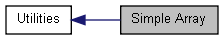## Simple Array [Utilities]

Collaboration diagram for Simple Array:### Functions

IUP_SDK_API Iarray * iupArrayCreate (int start_max_count, int elem_size)
IUP_SDK_API void iupArrayDestroy (Iarray *iarray)
IUP_SDK_API void * iupArrayGetData (Iarray *iarray)
IUP_SDK_API void * iupArrayReleaseData (Iarray *iarray)
IUP_SDK_API void * iupArrayInc (Iarray *iarray)
IUP_SDK_API void * iupArrayAdd (Iarray *iarray, int add_count)
IUP_SDK_API void * iupArrayInsert (Iarray *iarray, int index, int insert_count)
IUP_SDK_API void iupArrayRemove (Iarray *iarray, int index, int remove_count)
IUP_SDK_API int iupArrayCount (Iarray *iarray)

### Detailed Description

Expandable array using a simple pointer.
See iup_array.h

### Function Documentation

 IUP_SDK_API Iarray* iupArrayCreate ( int start_max_count, int elem_size )

Creates an array with an initial room for elements, and the element size. The array count starts at 0. And the maximum number of elements starts at the given count. The maximum number of elements is increased by the start_max_count, every time it needs more memory. Data is always initialized with zeros. Must call iupArrayInc, iupArrayAdd or iupArrayInsert to properly increase the number of elements.

 IUP_SDK_API void iupArrayDestroy ( Iarray * iarray )

Destroys the array.

 IUP_SDK_API void* iupArrayGetData ( Iarray * iarray )

Returns the pointer that contains the array.

 IUP_SDK_API void* iupArrayReleaseData ( Iarray * iarray )

Returns the pointer that contains the array, but also release it to be used elsewhere.

 IUP_SDK_API void* iupArrayInc ( Iarray * iarray )

Increments the number of elements in the array. The array count starts at 0. If the maximum number of elements is reached, the memory allocated is increased by the initial start count. Data is always initialized with zeros. Returns the pointer that contains the array.

 IUP_SDK_API void* iupArrayAdd ( Iarray * iarray, int add_count )

Increments the number of elements in the array by a given count. New space is allocated at the end of the array. If the maximum number of elements is reached, the memory allocated is increased by the given count. Data is always initialized with zeros. Returns the pointer that contains the array.

 IUP_SDK_API void* iupArrayInsert ( Iarray * iarray, int index, int insert_count )

Increments the number of elements in the array by a given count and moves the data so the new space starts at index. If the maximum number of elements is reached, the memory allocated is increased by the given count. Data is always initialized with zeros. Returns the pointer that contains the array.

 IUP_SDK_API void iupArrayRemove ( Iarray * iarray, int index, int remove_count )

Remove the number of elements from the array. Memory allocation remains the same.

 IUP_SDK_API int iupArrayCount ( Iarray * iarray )

Returns the actual number of elements in the array.